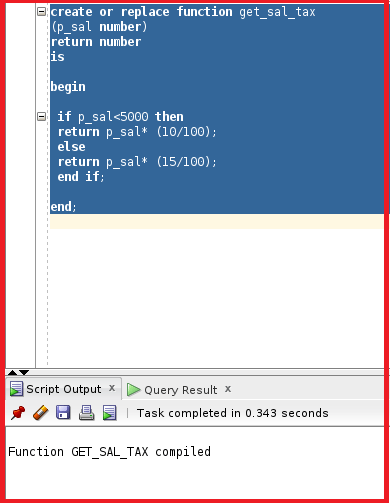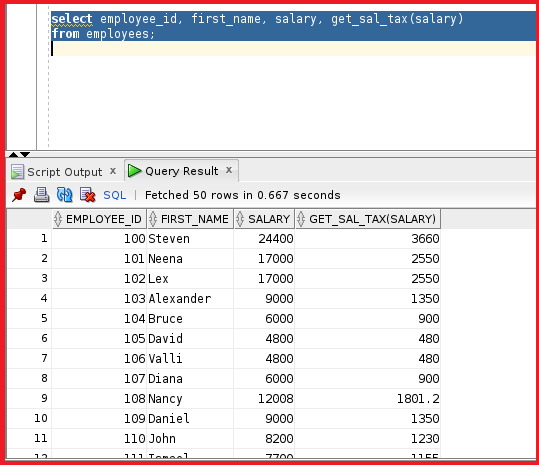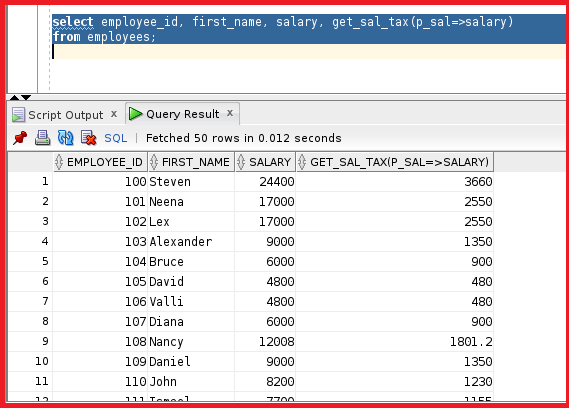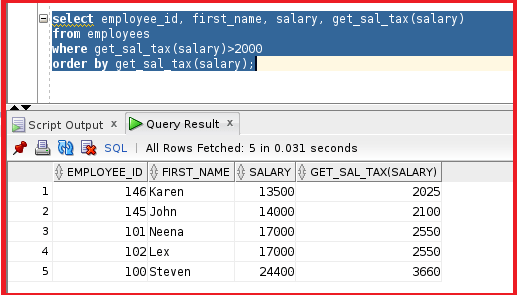# Advantages of User Defined Functions in Oracle

## Advantages of User-Defined Functions in Oracle

In this article, I am going to discuss the Advantages of User Defined Functions in Oracle with Examples. Please read our previous article where we discussed How to Create User Defined Functions in Oracle with Examples.

##### Advantages of User-Defined Functions in Oracle

We have already learned that we can use user-defined functions in SQL statements. But now let’s understand the advantages of using the user Defined functions.

##### Advantages of Functions in Oracle:
• Can extend SQL where activities are too compiled, too awkward, or unavailable with SQL. Sometimes we have very complex SQL codes that normally are unable to perform. So, we use the user-defined function in that situation.
• Can Increase efficiency when used in the WHERE clause to filter data, as opposed to filtering the data in the application.
• Can manipulate data values. We can use the user-defined functions in insert or update.
##### Calling User-Defined Functions in SQL Statements:

User-defined functions act like built-in single-row functions and can be used in:

• We can deal with the user-defined functions just like built-in functions like upper, lower, init char, in string, and substring.
• The SELECT list or clause of a query.
• Conditional expression of the WHERE AND HAVING clauses.
• The CONNECT BY, START WITH, ORDER BY, AND GROUP BY clauses of a query. This is the feature for Oracle queries.
• The VALUES clause of the INSERT statement.
• The SET clause of the UPDATE statement.

Now, let’s do some exercises about the user-defined functions. Let’s go ahead and create a function to calculate the tax on the salary. If the salary is greater than 5000 then the tax is 10 % or else the tax is 15%.

```create or replace function get_sal_tax
(p_sal number)
return number
is

begin

if p_sal<5000 then
return p_sal* (10/100);
else
return p_sal* (15/100);
end if;

end;
```

We have created a function with the name “get_sal_tax” where we are taking p_sal as IN parameter which also returns a number. The main logic of the function is if the p_sal is less than 5000 then return 10% of the p_sal value or else return 15% of the p_sal value. We are using the percentage as p_sal multiplying with 10 or 15 and dividing by 100 which finally gives the percentage of the p_sal variable. Let’s go ahead and compile this function.So, the function GET_SAL_TAX is now compiled. Let’s try to test the function by checking the percentage of each employee in the employees table.

Select employee_id, first_name, salary, get_sal_tax(salary) from employees;

Here we are trying to call the function in the select statement and passing salary as the IN parameter to the function GET_SAL_TAX. Now, let’s try to run this statement and see the percentage.We can see the GET_SAL_TAX column in the output. From the output, we can see the employee Steven has a salary of 24400 which is more than 5000 so his percentage is 15%. So, Steven’s tax as per our function calculation is 3660. So, as per the function, the salary 24400 is sent to the function as the IN parameter and then the salary is converted into tax and returned by the function.

We can use the user-defined functions in both SQL statements and select statements. I will use the same example and use the naming notation.

Select employee_id, first_name, salary, get_sal_tax(p_sal=>salary) from employees;

We are calling the function in the select statement and passing the parameter p_sal which takes salary as the value. Let’s try to execute this statement.As we can see the output is the same as the previous result. Let’s try something different.

Select employee_id, first_name, salary, get_sal_tax(salary) from employees where get_sal_tax(salary)>2000 order by get_sal_tax(salary);

So, we are filtering the tax which is more than 2000, and ordering by function.So, we can see only 5 employees whose tax in the salary is greater than 2000. This is how we perform user-defined functions in our Realtime based scenarios.

In the next article, I am going to discuss Restrictions When Calling Functions in Oracle with Examples. Here, in this article, I try to explain the Advantages of User Defined Functions in Oracle with Examples. I hope you enjoy this Advantages of User Defined Functions in Oracle article.